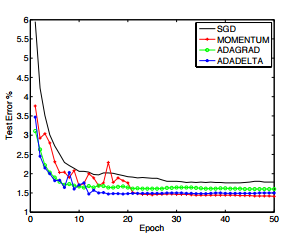This paper was done by Matthew D. Zeiler while he was an intern at Google.

### Introduction

The aim of many machine learning methods is to update a set of parameters $x$ in order to optimize an objective function $f(x)$. This often involves some iterative procedure which applies changes to the parameters, $\Delta{x}$ at each iteration of the algorithm. Denoting the parameters at the t-th iteration as $x_t$, this simple update rule becomes:

• $g_t$ is the gradient of the parameters at the t-th iteration
• $η$ is a learning rate which controls how large of a step to take in the direction of the negative gradient

### Purpose

The idea presented in this paper was derived from ADAGRAD in order to improve upon the two main drawbacks of the method:

1. the continual decay of learning rates throughout training
2. the need for a manually selected global learning rate.

• SGD: $\Delta{x\_t} = \rho{\Delta{x\_{t-1}}} - \eta{g\_t}$
• where $\rho$ is a constant controlling the decay of the previous parameter updates
• ADAGRAD: $\Delta{x\_t} = -{ {\eta} \over \sqrt{\sum\_{T=1}^t g\_{T}^2} }$
• ADADELTA: $\Delta{x\_t} = -{ RMS$\Delta{x}$\_{t-1} \over RMS$g$\_t}g\_t$ $RMS[g]\_t = \sqrt{E[g^2]\_t + \epsilon}$ $E[g^2]\_t = \rho{E[g^2]\_{t-1} } + (1-\rho)g_{t}^2$
• where a constant $\epsilon$ is added to better condition the denominator
• where $E[g^2]_t$ is expected value of gradient with power 2 at time t

### Result

Have tried ADADELTA and SGD. Although for each epoch ADADELTA takes longer time to compute, we just have to input (default value) $\rho = 0.95$ and $\epsilon = 1e^{-6}$ then it will learn very well. If use SGD, we have to fine tune the learning rate and the error rate is often bigger than ADADELTA.